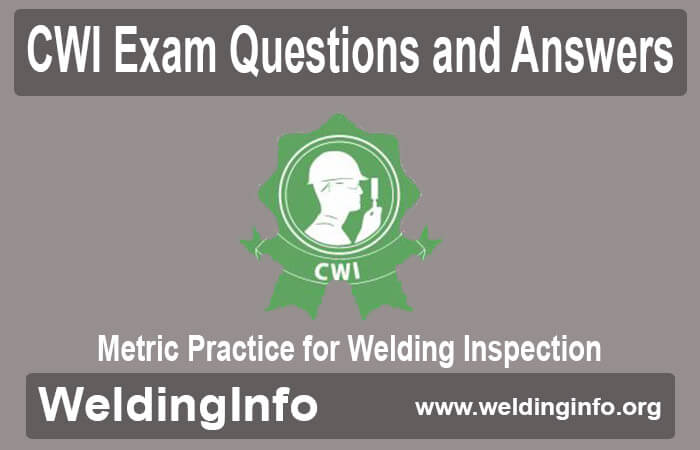# Metric Practice for Welding Inspection Q&A

CWI Exam has three parts i.e. Part A, Part B, and Part C. Each part has a specific syllabus and schedule. Many applicants prepare for the Certified Welding Inspector exam but all cannot pass it due to lack of proper study materials. It consists of AWS welding books, seminars, questions banks, etc. The following are questions based on the metric practice for welding inspection topic. Prospective applicants can learn these questions and answers for the CWI exam along with other welding tests.

## CWI Exam Questions (Metric Practice for Welding Inspection)

1. What is the value (in psi) of a material having a tensile strength of 700 MPa?
i) 1,015 psi
ii) 101,500 psi
iii) 101.50 psi
iv) 10.150 psi
v) 1,015 x 108 psi

Ans. (ii)
Explanation: conversion factor for MPa to psi is 1.450 x 102
700 MPa x 1.45 x 102 = 1,015.00 x 102 on the calculator
= 101,500 psi

2. Name the country which is not officially on the SI system?
i) Australia
ii) Japan
iii) United States
iv) Mexico
v) Britain

Ans. (iii)

3. What is the base unit ounce used to measure in the U.S. customary system?
i) Volume
ii) Mass
iii) All the above
iv) Distance

Ans. (iii)

4. The U.S. system of measurement is based on powers of 10.
i) False
ii) True

Ans. (i)

5. What is the base unit that is used to measure the pressure and tensile strength in the SI?
i) Pascal
ii) Newton
iii) Liter
iv) Hertz
v) Meter

Ans. (i)

6. How many kgs a 50.0 lb can of welding electrodes weighs?
i) 7 kg
ii) 227 kg
iii) 23,000 kg
iv) 25 kg
v) None of the above

Ans. (i)
Explanation: lbs to kg conversion factor is 0.454 then 0.454 x 50 = 22.7

7. When rounded to the nearest tenths, what is 4,532.182?
i) 4,532.2
ii) 4,530
iii) 4,532
iv) 4,532.1
v) 4,532.18

Ans. (i)

8. What is the value a material having a tensile strength of 8.5 x 104 psi in megapascals?
i) 5.86 x 103 MPa
ii) 5.9 x 106 MPa
iii) 586MPa
iv) All the above
v) 58 MPa

Ans. (iii)
Explanation: The conversion factor for psi to MPa is 6.895 x 10-3
So, 8.5 x 104 x 6.895 x 10-3 = 58. 6075 x 101 on the calculator
= 586 MPa

9. How long is a weld joint (measured and found to be 345 mm long) in terms of inches?
i) 8760
ii) 135
iii) 13.58 in.
iv) 13.0 in
v) 876

Ans. (iii)
Explanation: mm to inches conversion factor is 3.937 x 10-2
345 x 3.937 x 10-2 = 1358.265 x 10-2 inches on calculator

10. What is the wire feed speed that is measured to be 175 in/min?
i) 75 mmls
ii) 4 mm/s
iii) 0.070 m/s
iv) 70 mm/s
v) 0 mm/s

Ans. (v)
Explanation: inches/minute to mm/s conversion factor is 0.423
175 x 0.423 = 74.025 mm/s on calculator

11. What is s gas flow rate of 30 cfh in ℓ/min?
i) 64 ℓ/min
ii) 4 ℓ/min
iii) 14 ℓ/min
iv 640 ℓ/min
v) 140 ℓ/min

Ans. (iii)
Explanation: cfh to l/min conversion factor is 4.719 x 10-1
So, 30 x 4.719 x 10-1 = 14.157

12. The metric system or SI is far more complicated than the U.S. system.
i) False
ii) True

Ans. (i)

13. For linear measurement, which of the following are the proper base unit(s) in the U.S. system?
i) Foot
ii) Yard
iii) Mile
iv) Inch
v) All the above

Ans. (v)

14. According to AWS, what is the base unit for measuring mass in the SI system?
i) Liter
ii) Megapascal
iii) Kilogram
iv Meter
v) None of the above

Ans. (iii)

15. There are many U.S. industries that presently use SI.
i) False
ii) True

Ans. (ii)

16. The U.S. worker must know which measurement system for more effectiveness?
i) U.S. Customary
ii) Metric
iii) SI
iv) Above All

Ans. (iv)

17. AWS has mandated the requirement that the metric system is used.
i) False
ii) True

Ans. (i)

18. What is the designation the AWS has prepared a guide for aiding the transition to metrication?
i) A3.0
ii) D1.1
iii) A1.1
iv) Section VIII
v) None of the above

Ans. (iii)

19. A regulator indicates 50 psi of gas pressure. What is this in kPa?
i) 3.45 kPa
ii) 345 kPa
iii) 7.25 kPa
iv) 3,450 kPa
v) 725 kPa

Ans. (ii)
Explanation: psi to kPa conversion factor is 6.895
So, 50 x 6.895 = 345

20. What is the name of unit in which a deposition rate is measured in the U.S. and SI systems?
i) lb/hr
ii) kg/hr
iii) i and ii above
iv) kg
v) lb

Ans. (iii)

Thus, the above-mentioned CWI exam questions and answers are beneficial for applicants preparing for the CWI exam. These questions clear various welding concepts of students.